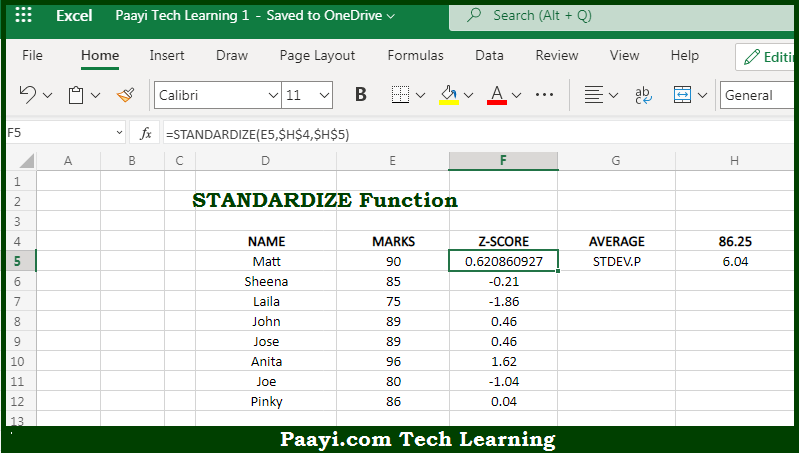# Learn How to Use Microsoft Excel STANDARDIZE Function

Written by | 0 Comments | 584 Views

In this article, you will learn how to use the Microsoft Excel STANDARDIZE function and its prime function in Microsoft Excel. You will also get to know the Microsoft Excel STANDARDIZE function return value and syntax with the help of some examples.

Microsoft Excel STANDARDIZE Function

The main purpose of the Microsoft Excel STANDARDIZE function is to calculate normalize value or z-score. That implies, with the help of STANDARDIZE function you can able to return a normalized value or z-score, based on the mean and standard deviation. A z-score, or standard score, can be understood as a way of standardizing scores on the same scale by dividing a score's deviation by the standard deviation in a data set. The result will be the standard score or a z-score. It is used to the number of standard deviations a given data point is from the mean So, with the help of STANDARDIZE function, you can able to calculate normalize value or z-score.

Return Value of STANDARDIZE Function

The return value will be the normalized value.

Syntax of STANDARDIZE Function

=STANDARDIZE(x, mean, standard-dev)

Where the arguments:

• x: This is the value to normalize.
• mean: This is the arithmetic mean of the distribution.
• standard-dev: This is the standard deviation of the distribution

How to Use Microsoft Excel STANDARDIZE Function?So we know that Microsoft Excel STANDARDIZE function you can able to calculate normalize value or z-score. That implies, with the help of STANDARDIZE function you can able to return a normalized value or z-score, based on the mean and standard deviation. A z-score, or standard score, can be understood as a way of standardizing scores on the same scale by dividing a score's deviation by the standard deviation in a data set. The result will be the standard score or a z-score. It is used to the number of standard deviations a given data point is from the mean So, with the help of STANDARDIZE function, you can able to calculate normalize value or z-score.